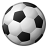# QlikView App Dev

Discussion Board for collaboration related to QlikView App Development.

Announcements
Join us at the Cloud Data and Analytics Tour! REGISTER TODAY
cancel
Showing results for
Search instead for
Did you mean:Not applicable

## variable evaluation

what is the difference between \$(Max(Year) and \$(=Max(Year)) ? I m really confused when to use which one ?

4 RepliesMVP & Luminary

Here a very good explanations:

The Magic of Variables

The Magic of Dollar Expansions

- MarcusSpecialist

Hi please read this post  by hicSpecialist II

Hi,

\$-sign expansion is a syntax form that allows a user to evaluate a variable, or an expression, and to replace the name or the expression with its result. The following is the list of some possible examples. While it’s not a complete list, it represents enough for the purpose of this analysis:

## \$-sign expansions using a variable:

When \$-sign expansion contains a variable, it will return the value of the variable at the time of the call. Changing the value of the variable can help make your application more flexible:

// In this example, the value is passed to the Database as a hard-coded value:
let var1 = 1000;
SQL SELECT * FROM Sales Where SalesAmount >= \$(var1);

// In this example, the chart expression is calculated dynamically, based on the value of the variable
SET var2 = ‘sum(Sales)’;
Chart Expression:                \$(var2)

## \$-sign expansions using formulas:

When \$-sign expansion contains a formula, the formula will get evaluated at the time of the call:

// In this example, the aggregation function is built based on the formula prior to being evaluated:
sum(\$(=’Expression’&vExprNumber))

// In this example, the formula is used as a part of a Set Analysis expression:
sum( {<Date={“>=\$(=Date(Today()-7))”}>} Sales)

## \$-sign expansion with parameters:

\$-sign expansion can contain a formula with one or more parameters that are specified as \$1, \$2, \$3:

// In this example, the formula can be modified dynamically based on the parameter:
SET vExpr = ‘sum(Expression’ & \$1 & ‘)’;
Chart Expression: \$(=\$(vExpr (1)))    – is equivalent to “sum(Expression1)”
Chart Expression: \$(=\$(vExpr (2)))    – is equivalent to “sum(Expression2)”Employee

The dollar sign expansion allows to bring values into a context, for example when you to put the value of a variable into a context. In the example below the MyVariable value is expanded into the expression.

=Sum(MyField) + \$(MyVariable)

In this setup the dollar sign expansion is always evaluated and expanded first, before the expression is evaluated. If MyVariable has a value of 5, the actually evaluated expression looks like below. A value has been expanded into the expression, and the expression is then evaluated based on the expanded value.

=Sum(MyField) + 5

In the same way you can use the dollar sign expansion to expand the result of an other expression. For example as in your exmaple whee you look for the max year. The equal sign inside the dollar expansion tells QlikView to evaluate the content of the dollar sign expansion as an expression. The result of the expression will then be expanded into the context where it is used.

\$(=Max(Year))

There must be a equal sign inside the dollar sign expansion if the content is expected to be evaluated. From that perspective your other example is an invalid statement.

\$(Max(Year) )